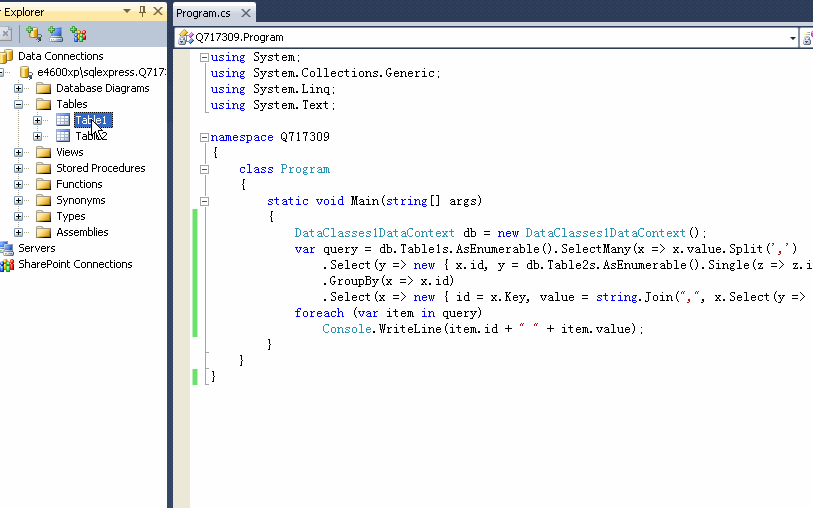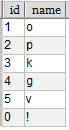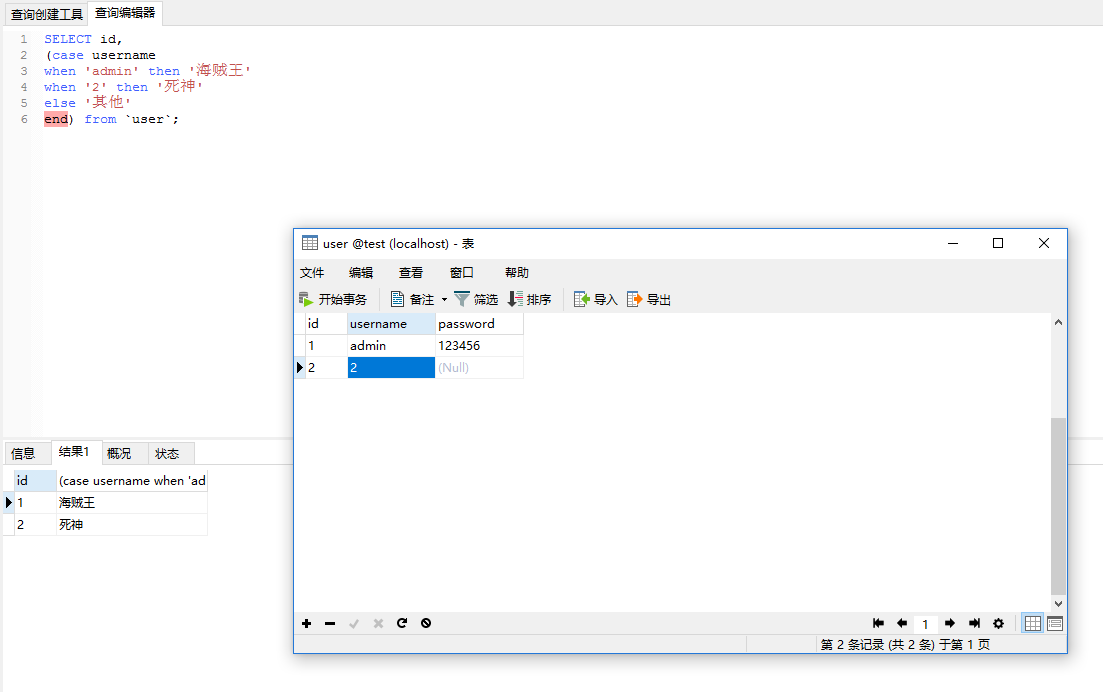``````1,2,3,4,5,6
1,2,5
``````
``````1  张三
2  王二
3  李四
4  麻子
5  小明
6  小红
``````

``````张三、王二、李四、麻子、小明、小红

``````

8个回答``````using System;
using System.Collections.Generic;
using System.Linq;
using System.Text;

namespace Q717309
{
class Program
{
static void Main(string[] args)
{
DataClasses1DataContext db = new DataClasses1DataContext();
var query = db.Table1s.AsEnumerable().SelectMany(x => x.value.Split(',')
.Select(y => new { x.id, y = db.Table2s.AsEnumerable().Single(z => z.id.ToString() == y.Trim()).text.Trim() }))
.GroupBy(x => x.id)
.Select(x => new { id = x.Key, value = string.Join(",", x.Select(y => y.y)) });
foreach (var item in query)
Console.WriteLine(item.id + " " + item.value);
}
}
}
``````

declare @S nvarchar(max) = '';
select @S = @S + [Name] + ','
from sj_enumvalue
where [Value] in (101,102,103)
select LEFT(@S, LEN(@S)-1);

decode 和case when then 都可以实现

create table zidian (id tinyint,name nvarchar(30))---放123456对应名称

CREATE FUNCTION [dbo].SplitStringToTable ,
@split VARCHAR(10)
)
RETURNS TABLE
AS
RETURN
( SELECT B.id
FROM ( SELECT [value] = CONVERT(XML , '' + REPLACE(@str , @split , '')
+ '')
) A
OUTER APPLY ( SELECT id = N.s.value('.' , 'varchar(100)')
FROM A.[value].nodes('/s') N ( s )
) B
)

declare @i as nvarchar(max)=''
select @i=@i+b.name+',' from zidian b left join (select dbo.SplitStringToTable('1,2,3,4,5,5,3,2',',') a on a.id=b.id
set @i=replace((@i+','),',,','')
select @i

declare @i as nvarchar(max)=''
select @i=@i+b.name+',' from zidian b cross apply (select * from dbo.SplitStringToTable('1,2,3,4,5,5,3,2',',')) a where a.id=b.id
set @i=replace((@i+','),',,','')
select @i

create table zifuchuan (c nvarchar(500))---字符串表
update zifuchuan set c=c+',0'---分行标识为0，且对应字典上为0字符加上!号对应关系declare @i as nvarchar(max)=''
select @i=@i+b.name+',' from zidian b cross apply (select id from zifuchuan ab cross apply dbo.SplitStringToTable(ab.c,',') abc) a where a.id=b.id
select * from dbo.SplitStringToTable(replace(@i+';',',!,;',''),',!,')

Select decode（columnname，值1,翻译值1,值2,翻译值2,...值n,翻译值n,缺省值）
from talbename

·含义解释：
decode(条件,值1,翻译值1,值2,翻译值2,...值n,翻译值n,缺省值)的理解如下：
if （条件==值1）
then
return(翻译值1)
elsif （条件==值2）
then
return(翻译值2)
......
elsif （条件==值n）
then
return(翻译值n)
else
return(缺省值)
end ifhometing218 回复zhangqwe886: 用于开发的话,,两种方式;1:sql语句,但是这样的话case when得根据值来,有多少个值就需要多少个;2:通过代码.查询出来后循环去设置对应的值hometing218 回复zhangqwe886: 可以啊.看自己的需求..根据需求去替换